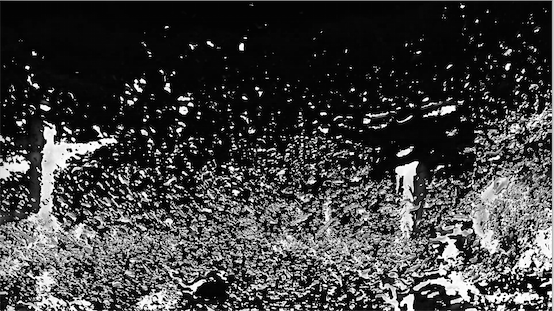# Image Subtraction Using Pillow

## Overview:

• Subtracting an image from another image results in an image with the differences between the two.

• When an Image I2 is subtracted from another Image I1 the resultant image Io will have its pixel values given by

pIo(x,y) = pI1(x,y) – pI2(x,y)

• The example Python program works on two digital images taken on a rainy day as an automobile moves by. The images are probably microseconds apart having very few differences among them.When subtracted, the differences are apparent - the lamp post on the left hand side, few splashed water drops and the water drops on the bottom portion of the windshield.

• Image subtraction has its applications in variety of fields including Astrophotography and Remote sensing.

• Digital Subtraction is a widely used technique in Medical Imaging as in the case of Digital Subtraction Angiography and studies involving disease prognosis.

## Example:

 # Python example program for image subtraction from PIL import Image import numpy as np   # Paths of two image frames image1Path = "./windshield1.jpg"; image2Path = "./windshield2.jpg";   # Open the images image1     = Image.open(image1Path); image2     = Image.open(image2Path);   # Get the image buffer as ndarray buffer1    = np.asarray(image1); buffer2    = np.asarray(image2);   # Subtract image2 from image1 buffer3    = buffer1 - buffer2;   # Construct a new Image from the resultant buffer differenceImage     = Image.fromarray(buffer3);   # Display all the images including the difference image image1.show(); image2.show(); differenceImage.show();

## Output:

### Input Image 2:### Image1 - Image2: